Rain Cloud Plots using Half Violin Plot with jittered data points in R

• Last Updated : 28 Nov, 2021

In this article, we will discuss how to create Rain Cloud Plots using Half Violin Plot with jittered data points in R

Raincloud plots or Half Violin plots are basically used to visualize the distribution and the overall summary of the data at the same time. This plot is a combination of half violin plots with jittered points on top, boxplots and can be further enhanced by adding central measures of tendency, quartile ranges, etc.

Dataset Used

Here let’s use the “iris” dataset. This is an in-built dataset in R.

R

Output: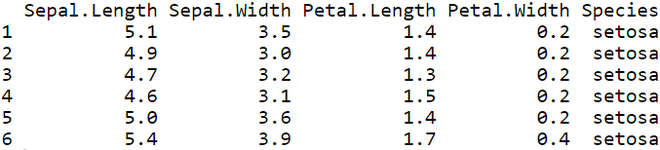Plotting a Raincloud using ggplot

Example 1: Simple Raincloud plot

Let’s plot a Raincloud/Half Violin plot for Species vs Sepal Length of iris dataset.

R

 # Loading the packages library("ggplot2")library("plyr")  # load the iris datadf <- iris   # Code to plot a raincloudggplot(df, aes(Species, Sepal.Length, fill=Species)) +geom_flat_violin() +coord_flip() +theme(legend.position = "none")

Output: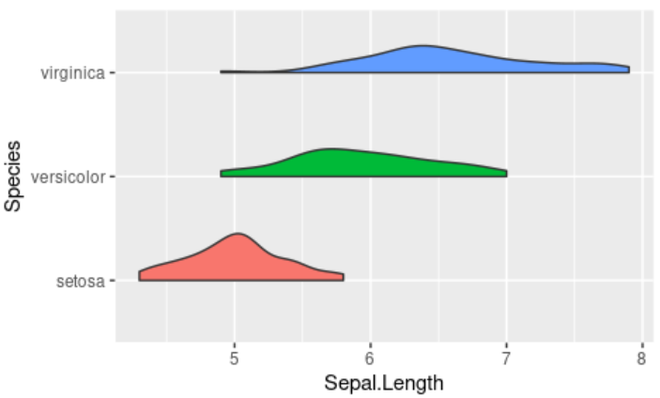Example 2: Raincloud with jittered data points on top

Let’s check out how to add jittered data points on top of the Raincloud plot using the geom_jitter( ) function.

R

 # Loading the packages library("ggplot2")library("plyr")  # load the iris datadf <- iris   # Code for Raincloud plot with jittered data pointsggplot(df, aes(Species, Sepal.Length, fill=Species)) +geom_flat_violin() +coord_flip() + geom_jitter(alpha = 0.5,width = 0.15)+theme(legend.position = "none")

Output: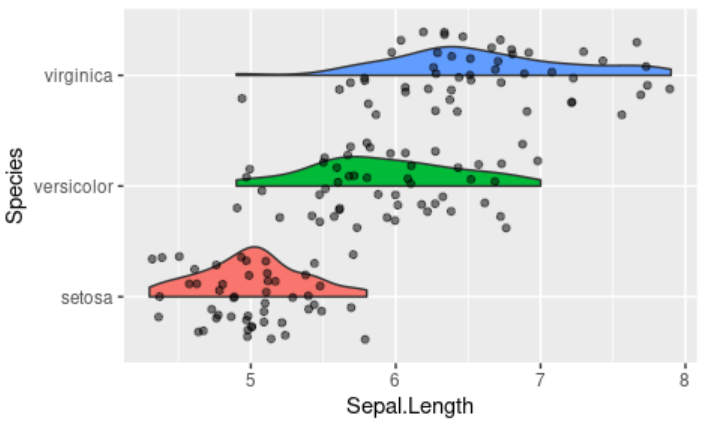Example 3: Positioning the Raincloud plot

Let’s see how to adjust the position of the Half violin plots with respect to the jittered data points.

R

 # Loading the packageslibrary("ggplot2")library("plyr")  # load the iris datadf < - iris  # Code for positioning the raincloud plotggplot(df, aes(Species, Sepal.Length, fill=Species)) +geom_flat_violin(position=position_nudge(x=.2, y=0)) +coord_flip() + geom_jitter(alpha=0.5, width=0.15, aes(color=Species)) +theme(legend.position="none")

Output: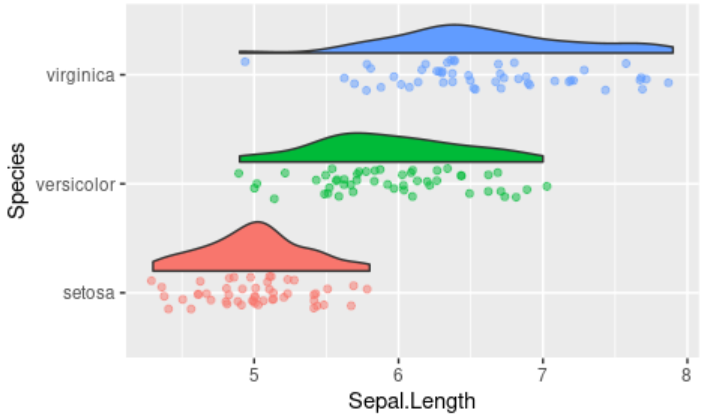Example 4: Raincloud Plot with Boxplots

Let’s see how to plot a Boxplot alongside the half violin plot in order to get additional insights of the features.

R

 # Loading the packageslibrary("ggplot2")library("plyr")  # load the iris datadf < - iris  # Code to plot Raincloud and boxplot ggplot(df, aes(Species, Sepal.Length, fill=Species)) +geom_flat_violin(position = position_nudge(x = .2, y = 0)) +coord_flip() + geom_jitter(alpha = 0.5,width = 0.15) +theme(legend.position = "none") +geom_boxplot( width = .25,  outlier.shape = NA )

Output: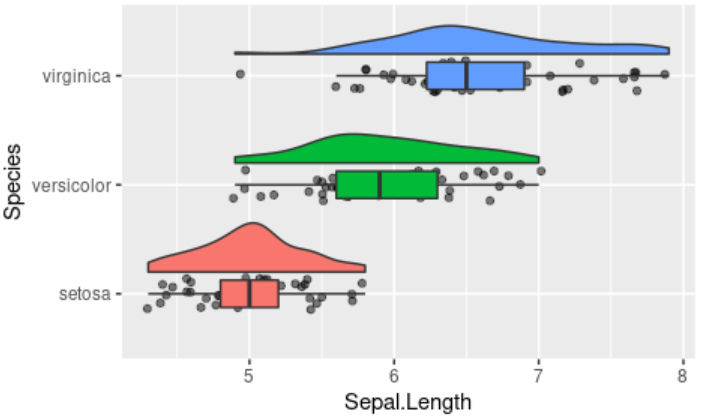My Personal Notes arrow_drop_up